# 8+ Sample Simple Metric Conversion Chart Templates

A simple metric system conversion chart is a mighty useful item in our day to day lives. On a regular basis, we have to convert between various units of weight, height, length and so on. Such conversions may be done mentally, but that vastly increases chances of errors. Instead, you can just opt for the Metric Conversion Chart Templates that are available online. These are easy to use and are at your disposal whenever you need them.

## Simple Metric Conversion Chart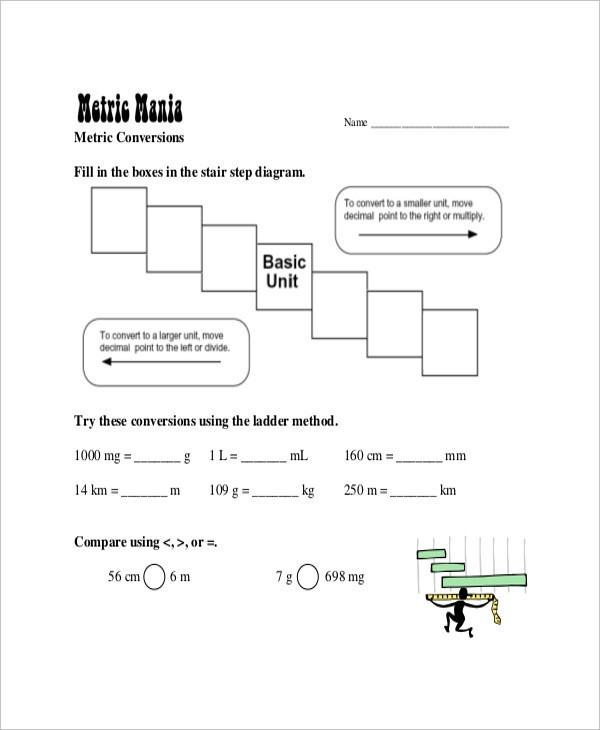sciencespot.net

The simple metric system conversion Chart Templates can be used in a versatile fashion. If you need to convert an SI unit into another unit or vice versa, you can make use of this chart.

## Simple Metric Weight Conversion Chart Template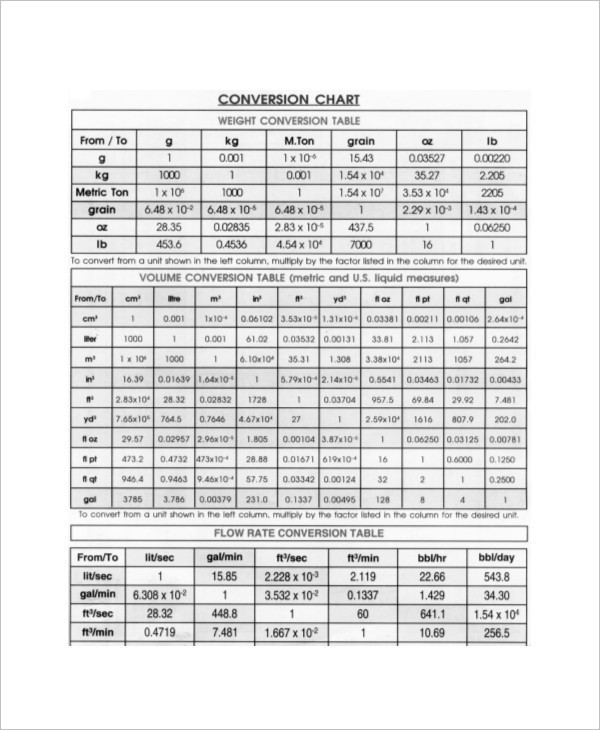blulux.com

This simple metric weight conversion chart has been reserved specifically for unit conversions of weight. For instance, if you need to convert between kilogram, gram, milligram or even centigram, then you can use this chart.

## Simple Metric Conversion Chart for Length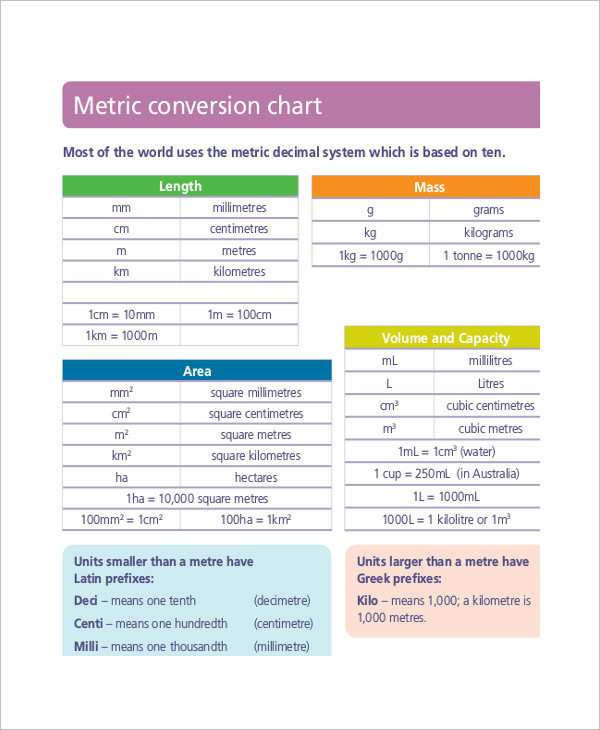det.nsw.edu.au

This simple metric conversion chart for length allows you to convert between the various units of length like meter, kilometer and so on. The chart may be used to calculate distances or lengths.

## Simple Metric Conversion Chart in Excel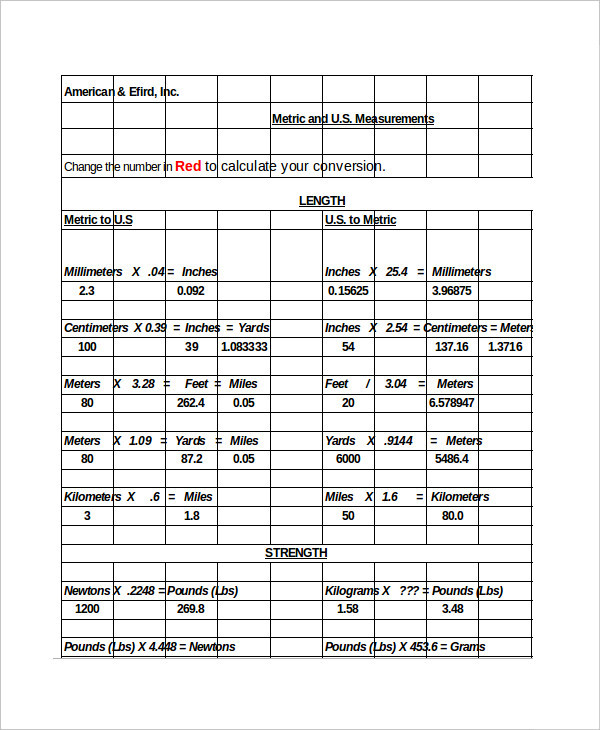amefird.com

If you are converting between units of different kinds, then excel would be your best shot. The simple metric conversion chart in excel allows you to organize your data in the form of neat rows and columns.

## Simple Imperial or Metric Conversion Chart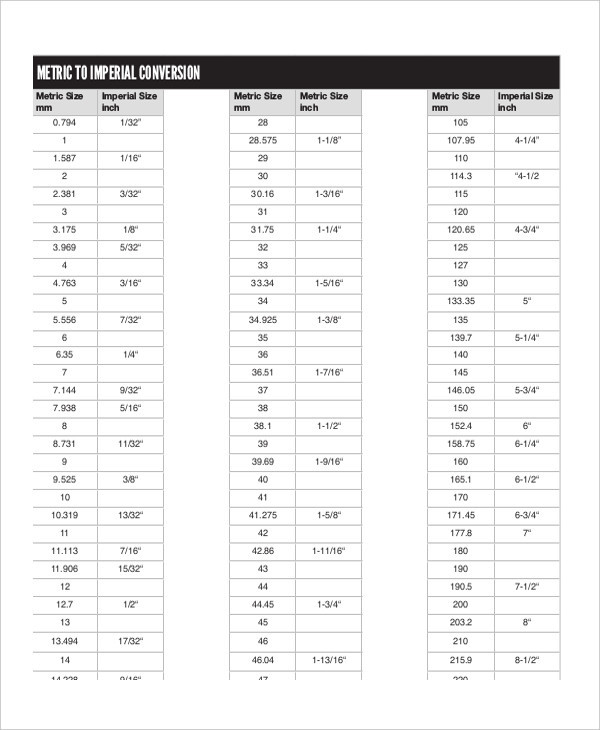interlloy.com.au

Here, imperial refers to the standard units accepted in the US and the conversions between such units and those accepted universally. For that, you can use this simple imperial-metric conversion chart.

## Simple Conversion Chart For Metric System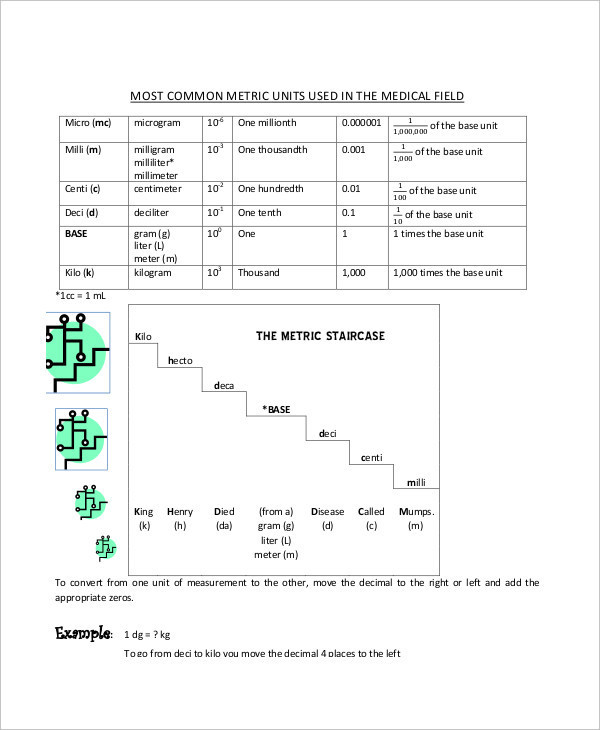laniertech.edu

## Simple Height & Weight Metric Conversion Chart Template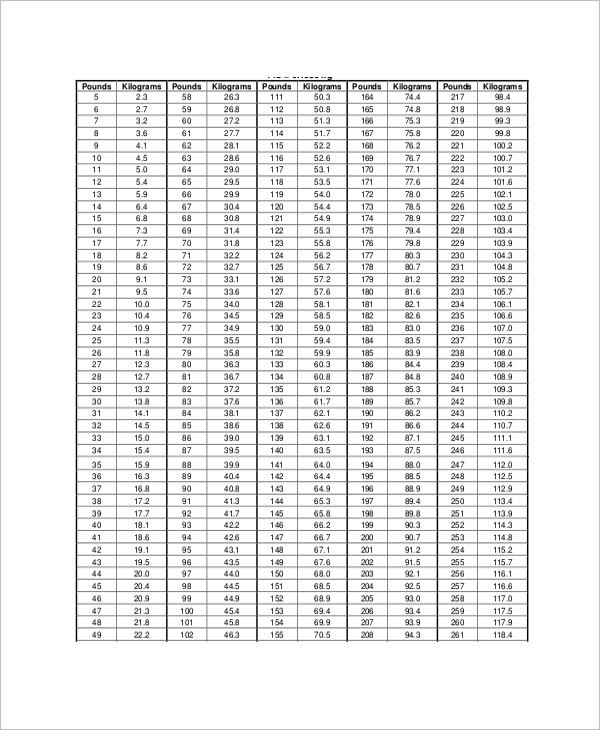calgarylabservices.com

## Simple Metric Unit Conversion Chart Example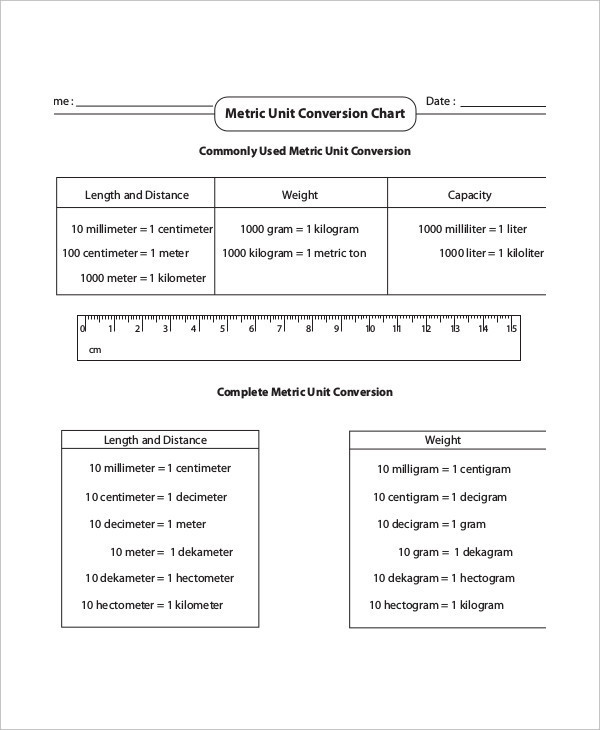mathworksheets4kids.com

### > How can you use the Simple Metric Conversion Charts?

As the very name suggests, the metric conversion charts here are extremely easy to use. The charts allow you to insert your data into the chart and then convert it into your desired unit. For example, if you are calculating distances, then you can convert between meter and kilometer or centimeter and millimeter since these happen to be the most commonly used units. Also, there are different groups of accepted units, including the SI system. In our day to day life, we are often required to convert between groups. In such cases, one may use these simple metric conversion charts. You can also see Normal Height And Weight Chart Templates.

### > What are the benefits of Simple Metric Conversion Charts?

The simple metric conversion charts here come with a large number of benefits. Some of them are as follows:

• The metric system conversion charts allow you to convert between the different groups of units including the International System of Units (SI system) and the commonly used units.
• The charts would save you a lot of time and energy since you wouldn’t have to do long calculations yourself.
• Also, the charts come in neat and well-organized layouts. That allows you to carry out as many calculations as you want.
• The charts would help you avoid silly mistakes in calculations as well. You can also see Metric System Conversion Chart Templates.

Converting between various units can be pretty complicated if you aren’t all that thorough with the procedure. However, these metric conversion charts can come to your rescue. These charts would allow you to convert between the various units of length, weight, height and so on. Also, the charts come in excel or other easy to use formats.